NameFlagsCard.TypeDescription & Constraints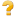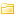Extension 0..*ExtensionAssociated insurance coverage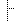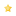extension 0..0url 1..1uri"http://hl7.org/fhir/StructureDefinition/request-insurance"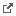value[x] 1..1Reference(Coverage | ClaimResponse)Value of extensionDocumentation for this format
<status value="active"/> <experimental value="false"/> <date value="2017-12-06"/> <publisher value="HL7 International / FHIR Infrastructure"/> <contact> <telecom> <system value="url"/> <value value="http://www.hl7.org/Special/committees/fiwg"/> </telecom> </contact> <description value="Insurance plans, coverage extensions, pre-authorizations and/or pre-determinations that may be relevant in delivering the requested service."/> <jurisdiction> <coding> <system value="http://unstats.un.org/unsd/methods/m49/m49.htm"/> <code value="001"/> </coding> </jurisdiction> <fhirVersion value="5.0.0"/> <mapping> <identity value="v2"/> <uri value="http://hl7.org/v2"/> <name value="HL7 v2 Mapping"/> </mapping> <mapping> <identity value="rim"/> <uri value="http://hl7.org/v3"/> <name value="RIM Mapping"/> </mapping> <kind value="complex-type"/> <abstract value="false"/> <context> <type value="element"/> <expression value="NutritionOrder"/> </context> <type value="Extension"/> <baseDefinition value="http://hl7.org/fhir/StructureDefinition/Extension"/> <derivation value="constraint"/> <snapshot> <element id="Extension"> <path value="Extension"/> <short value="Associated insurance coverage"/> <definition value="Insurance plans, coverage extensions, pre-authorizations and/or pre-determinations that may be relevant in delivering the requested service."/> <min value="0"/> <max value="*"/> <base> <path value="Extension"/> <min value="0"/> <max value="*"/> </base> <constraint> <key value="ele-1"/> <severity value="error"/> <human value="All FHIR elements must have a @value or children"/> <expression value="hasValue() or (children().count() > id.count())"/> <source value="http://hl7.org/fhir/StructureDefinition/Element"/> </constraint> <constraint> <key value="ext-1"/> <severity value="error"/> <human value="Must have either extensions or value[x], not both"/> <expression value="extension.exists() != value.exists()"/> <source value="http://hl7.org/fhir/StructureDefinition/Extension"/> </constraint> <isModifier value="false"/> <mapping> <identity value="v2"/> <map value="IN1/IN2"/> </mapping> <mapping> <identity value="rim"/> <map value=".outboundRelationship[typeCode=COVBY].target"/> </mapping> </element> <element id="Extension.id"> <path value="Extension.id"/> <representation value="xmlAttr"/> <short value="Unique id for inter-element referencing"/> <definition value="Unique id for the element within a resource (for internal references). This may be any string value that does not contain spaces."/> <min value="0"/> <max value="1"/> <base> <path value="Element.id"/> <min value="0"/> <max value="1"/> </base> <type> <extension url="http://hl7.org/fhir/StructureDefinition/structuredefinition-fhir-type"> <valueUrl value="id"/> </extension> <code value="http://hl7.org/fhirpath/System.String"/> </type> <condition value="ele-1"/> <isModifier value="false"/> <isSummary value="false"/> <mapping> <identity value="rim"/> <map value="n/a"/> </mapping> </element> <element id="Extension.extension"> <path value="Extension.extension"/> <slicing> <discriminator> <type value="value"/> <path value="url"/> </discriminator> <description value="Extensions are always sliced by (at least) url"/> <rules value="open"/> </slicing> <short value="Extension"/> <definition value="An Extension"/> <min value="0"/> <max value="0"/> <base> <path value="Element.extension"/> <min value="0"/> <max value="*"/> </base> <type> <code value="Extension"/> </type> <constraint> <key value="ele-1"/> <severity value="error"/> <human value="All FHIR elements must have a @value or children"/> <expression value="hasValue() or (children().count() > id.count())"/> <source value="http://hl7.org/fhir/StructureDefinition/Element"/> </constraint> <constraint> <key value="ext-1"/> <severity value="error"/> <human value="Must have either extensions or value[x], not both"/> <expression value="extension.exists() != value.exists()"/> <source value="http://hl7.org/fhir/StructureDefinition/Extension"/> </constraint> <isModifier value="false"/> <isSummary value="false"/> </element> <element id="Extension.url"> <path value="Extension.url"/> <representation value="xmlAttr"/> <short value="identifies the meaning of the extension"/> <definition value="Source of the definition for the extension code - a logical name or a URL."/> <comment value="The definition may point directly to a computable or human-readable definition of the extensibility codes, or it may be a logical URI as declared in some other specification. The definition SHALL be a URI for the Structure Definition defining the extension."/> <min value="1"/> <max value="1"/> <base> <path value="Extension.url"/> <min value="1"/> <max value="1"/> </base> <type> <extension url="http://hl7.org/fhir/StructureDefinition/structuredefinition-fhir-type"> <valueUrl value="uri"/> </extension> <code value="http://hl7.org/fhirpath/System.String"/> </type> <fixedUri value="http://hl7.org/fhir/StructureDefinition/request-insurance"/> <isModifier value="false"/> <isSummary value="false"/> <mapping> <identity value="rim"/> <map value="N/A"/> </mapping> </element> <element id="Extension.value[x]"> <path value="Extension.value[x]"/> <short value="Value of extension"/> <definition value="Value of extension - must be one of a constrained set of the data types (see [Extensibility](http://hl7.org/fhir/R5/extensibility.html) for a list)."/> <min value="1"/> <max value="1"/> <base> <path value="Extension.value[x]"/> <min value="0"/> <max value="1"/> </base> <type> <code value="Reference"/> <targetProfile value="http://hl7.org/fhir/StructureDefinition/Coverage"/> <targetProfile value="http://hl7.org/fhir/StructureDefinition/ClaimResponse"/> </type> <condition value="ext-1"/> <constraint> <key value="ele-1"/> <severity value="error"/> <human value="All FHIR elements must have a @value or children"/> <expression value="hasValue() or (children().count() > id.count())"/> <source value="http://hl7.org/fhir/StructureDefinition/Element"/> </constraint> <isModifier value="false"/> <isSummary value="false"/> <mapping> <identity value="rim"/> <map value="N/A"/> </mapping> </element> </snapshot> <differential> <element id="Extension"> <path value="Extension"/> <short value="Associated insurance coverage"/> <definition value="Insurance plans, coverage extensions, pre-authorizations and/or pre-determinations that may be relevant in delivering the requested service."/> <min value="0"/> <max value="*"/> <mapping> <identity value="v2"/> <map value="IN1/IN2"/> </mapping> <mapping> <identity value="rim"/> <map value=".outboundRelationship[typeCode=COVBY].target"/> </mapping> </element> <element id="Extension.extension"> <path value="Extension.extension"/> <max value="0"/> </element> <element id="Extension.url"> <path value="Extension.url"/> <fixedUri value="http://hl7.org/fhir/StructureDefinition/request-insurance"/> </element> <element id="Extension.value[x]"> <path value="Extension.value[x]"/> <min value="1"/> <type> <code value="Reference"/> <targetProfile value="http://hl7.org/fhir/StructureDefinition/Coverage"/> <targetProfile value="http://hl7.org/fhir/StructureDefinition/ClaimResponse"/> </type> </element> </differential> </StructureDefinition>